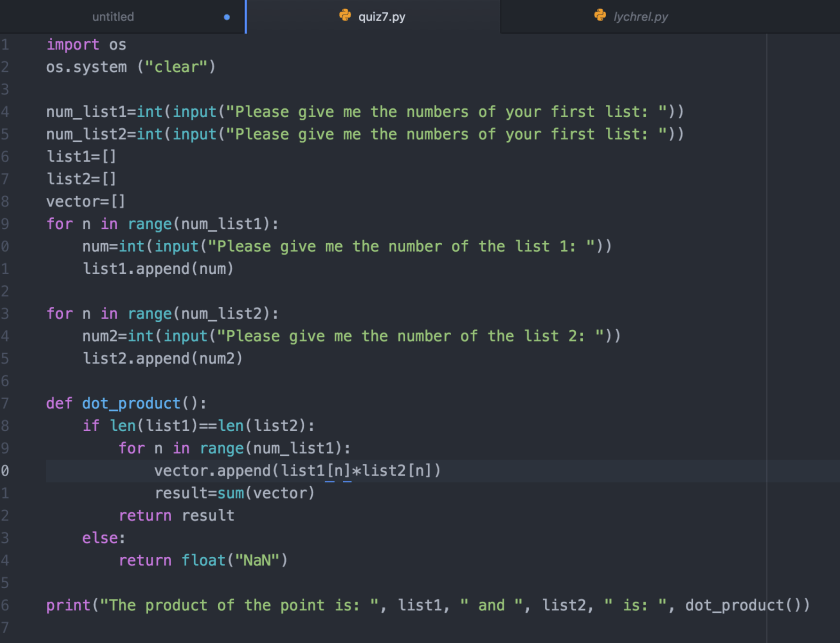## The Quiz

In mathematics, the dot product, or scalar product (or sometimes inner product in the
context of Euclidean space), is an algebraic operation that takes two equal-length
sequences of numbers (usually coordinate vectors) and returns a single number.

Algebraically, it is the sum of the products of the corresponding entries of the two
sequences of numbers

## What to Do

Create a function called dot_product that receives two lists of numbers (say list1 and
list2). The function returns what is the dot product of the two lists.

For full marks, if the lists are not the same size, then the function should return the
special value of NaN (which represents not a number).

• For Python, you can create this value with the expression: float(‘NaN’)
• For C++, check here: http://www.cplusplus.com/reference/cmath/nan-function/

Example. If the input is [2,4,5,6] and [1,2,3,4] the result will be 49 since (2*1)+(4*2)+(5*3)+(6*4) = 49

So this was me trying to do this quiz

**Thanks to this program we can make the product of two lists, it’s very simple, you only multiply each number of the list one by one, but as always in life, there’s one exception, if the size of the list is not equal, then you can’t make the product of the lists, so we have to make a conditional that makes sure to compare the size of the lists and it tells you if there are equals or not, to know the size of the list we can use len.

So here’s the screenshot of the program